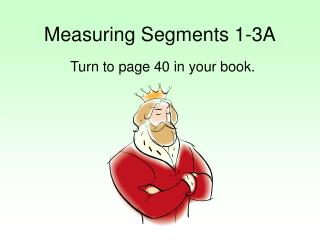DownloadDownload PresentationMeasuring Segments 1-3A

# Measuring Segments 1-3A

Télécharger la présentation## Measuring Segments 1-3A

- - - - - - - - - - - - - - - - - - - - - - - - - - - E N D - - - - - - - - - - - - - - - - - - - - - - - - - - -
##### Presentation Transcript

1. Measuring Segments 1-3A Turn to page 40 in your book.

2. Find the length of segments To find the length of a segment, first find the difference of the coordinates of its endpoints. Then since distance is always positive, take the absolute value of the result. A B C −10 −5 0 5 10 Find the length of segments

3. Far From It • Look at the vertical segment AB on the coordinate plane. What is the ordered pair for point A? Point B? What is AB? • What do you notice about the x-coordinates for the endpoints of AB? Their y-coordinates? • Now find the length of EF. A E F B

4. Distance Formula In Algebra, you used the distance formula to find the distance between any two points on a coordinate plane. A C B Find the length of AB, BC, and AC.

5. Find the length of RS, ST, and TR to the nearest tenth in the figure. R S T RS=5.1, ST=7.1, TR=6

6. Find the Length Find the length of XY, XZ, and YZ on a number line if the coordinate of X is −1.3, the coordinate of Y is −4, and the coordinate of Z is 2.7. −10 −5 0 5 10

7. Assignment p. 42, 1-10, 17-19, 23-29